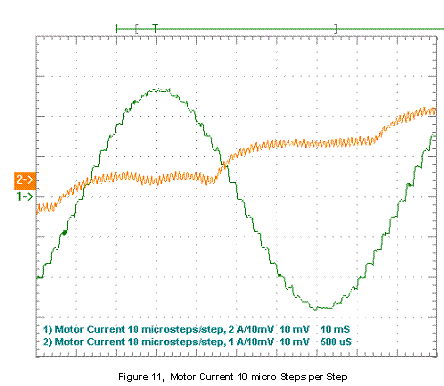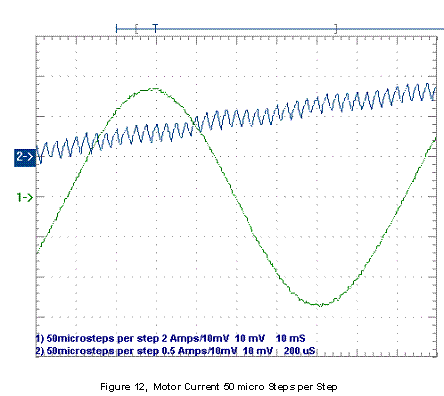# Microstepping Motor Drive 2002 - 2004

For the Mill Drill Retrofit project I designed and built my own current mode microstepping drives. The input to the drive is a step and direction command. After the drive was built an article was written.

by

John Figie

Dave Wilson

Copyright John Figie, Dave Wilson 2004

Since their birth in the early 1960’s as a replacement for servos in computer peripheral equipment, stepper motors have enjoyed extensive use in applications requiring tight position control at an affordable price.  Two reasons attributing to the popularity of stepper-based designs are their ability to achieve accurate position control without the requirement of position feedback (open-loop control), and the fact that they can be driven by squarewaves (figure 1), which can easily be generated by a digital controller.  However, make no mistake, stepper motors are NOT “digital motors”, as they have sometimes been called.  Such oversimplification can often lead to an underestimation of the complex nature of these electromechanical devices.  In fact, due to the inherent low damping factor associated with steppers, their control is often more difficult than with other motors, as resonance and acoustic noise problems frequently plague stepper designs.  To mitigate these problems, steppers are often driven with sinewaves instead of squarewaves (figure 2), which also results in tighter accuracy and precision control compared to squarewaves.

Several integrated devices available today are capable of directly driving a stepper, where the sinewave “microstepping” can be controlled with simple logic inputs.  However, many applications still require a processor for complex velocity profile generation, human interfacing, system communications, etc.  The application discussed here details the specific implementation of a current mode  microstepped control system built around Motorola’s MC68HC908MR16 microcontroller (figure 3).  The 6 channel PWM module, 10-bit ADC, and 16 bit timers on the ‘MR16 make it ideally suited for controlling a stepper in this fashion (figure 4).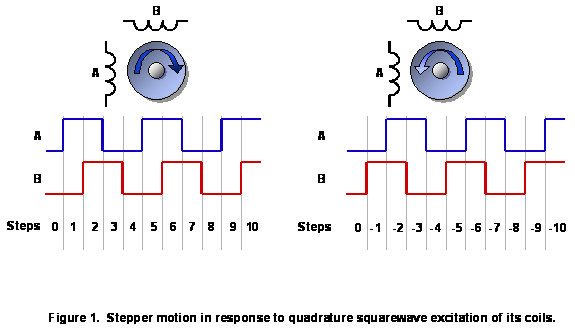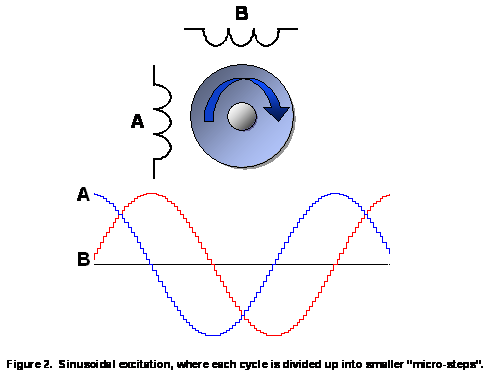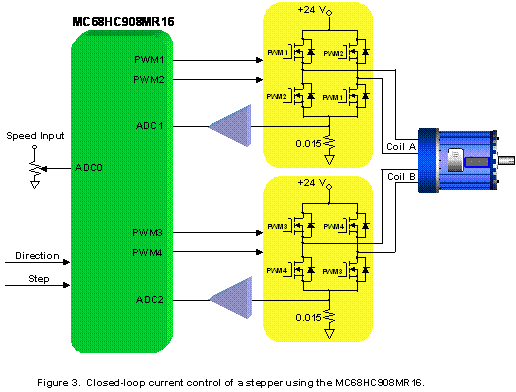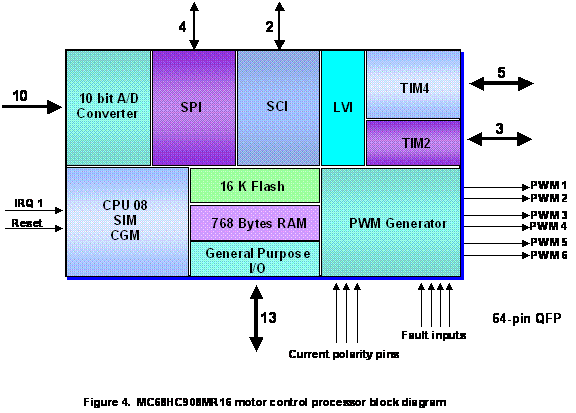A lookup table of 8-bit values constituting a sinewave is stored in Flash memory, and values are fetched from the table via a pointer, which scrolls through the table at a rate determined by the desired stepper speed (figure 5).  In this application, the pointer value is updated and a new wavetable value is fetched every 100 uS.  If the desired stepper speed is high, the pointer increment will be large, and some points in the table will be skipped.  In other words, when the stepper is in motion, it is not necessary to hit EVERY microstep value when synthesizing the sinewaves.  On the other hand, if the desired stepper speed is low, then the pointer increment will be small.  In fact, if the increment is small enough, it will result in the pointer fetching the same waveform value that was fetched last time.  In order to maintain good frequency resolution over the entire speed range, the pointer math is performed using 24-bit arithmetic, where 16 of the bits represent the fractional component.  After the pointer update operation is complete, only the integer portion of the pointer is used to fetch the desired value from the wavetable.  Also, modulo addressing must be performed on the pointer when it reaches one end of the table to insure that it wraps to the other end.   The size of the table should contain enough waveform points to provide smooth operation of the motor.  It may also be desirable to choose a size that results in a round number of microsteps per motor revolution.  For example, on a 200 step per revolution motor, if each step (90 degrees of the waveform) is divided into 10 microsteps, then the number of points for one complete sinewave cycle will be 40 points, resulting in 2000 microsteps per revolution.

As seen in figure 2, both a sine wave and cosine wave must be synthesized.  Instead of maintaining two separate wavetables, the same pointer is used to reconstruct both waveforms by simply adding the equivalent of 90 degrees, and performing a second fetch from the table.  To make the stepper go in the reverse direction, the pointer increment is simply subtracted during each update interval instead of added.  By running time “backwards” in this fashion, the phase relationship between the two generated waveforms is reversed.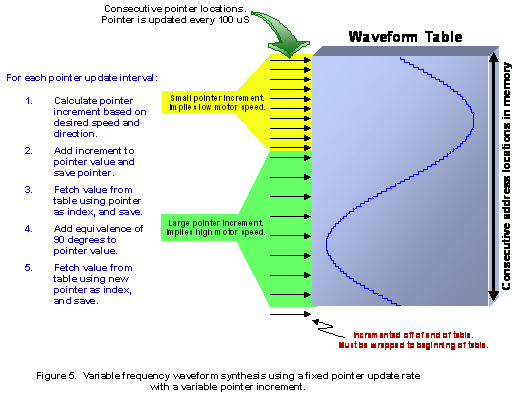Once the sinewaves have been generated, there are several ways they can be used to drive a stepper.  While some designs simply modulate the motor’s voltage with the sinewaves, most true microstepped designs directly control the motor’s current.  This yields better microstepping accuracy, and also results in better immunity to system imperfections, such as power supply perturbations, coil crosstalk, and changes in coil resistance over operating temperature.  However, this technique does utilize closed-loop feedback control, which is slightly more complex than simple voltage control.

Fortunately, upon inspection of the transfer function for this application from the H-bridge PWM input to the resulting motor current output, only one pole is found, which is the electrical pole caused by the stepper winding inductance and resistance.  This is endemic to current mode controllers found in many power supplies and motor drives, and suggests a design strategy for simple proportional controller that does not require a phase lead compensator.  Figure 6 shows the resulting block diagram of the entire closed-loop system for one motor phase.  To control both phases of the motor, two such loops must exist.  The resulting magnitude and phase plots for the open-loop transfer function are shown in figure 7.  As can be seen, the single pole of the motor’s voltage-to-current transfer function results in a phase margin of about 90 degrees, which is more than adequate to ensure stable operation.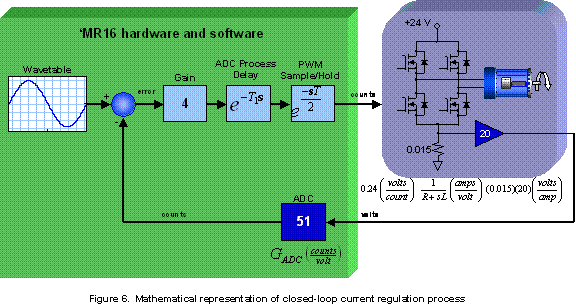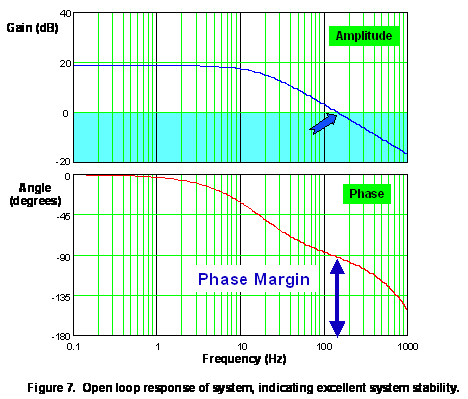Figure 8 shows a timing diagram of the switching waveforms on the H-bridge, and resulting shunt current.  Switches in the H-bridge are opened and closed as diagonal pairs, with separate PWM outputs from the MR16 controlling each diagonal pair.  For every PWM cycle, switches 1 and 4 are closed at the start of the cycle, but then open up to allow switches 2 and 3 to close for the last portion of the cycle.  This results in what is known as “bipolar PWMs”, since a high frequency AC voltage is impressed upon the motor windings.  Generally the PWM frequency should be chosen so that this current ripple is small with respect to the maximum rated motor current.  This switching technique also results in fast decay of the current, since the power supply is included in the current commutation path, which improves high-speed performance of the motor.  Immediately after the turn-off of any switch pair, the MR16 PWM module automatically inserts a “dead-time” in the waveforms before turning on the other switch pair in order to prevent “shoot-through” currents.

When switches 1 and 4 are on for more than 50% of the time, the steady state motor current is positive, and when they are on for less than 50% of the time, the steady state motor current is negative.  The motor inductance filters the PWM voltage to generate a motor current that ramps up and down through the PWM cycle.  A sense resistor is placed in the ground return path of the H-bridge to measure this motor current.  As the PWM signals toggle, a bipolar voltage is generated across the sense resistor.  However, the bias network on the op-amp input (figure 8) results in a unipolar op-amp output signal, where zero motor current corresponds to 2.5V (half of the ADC conversion range).  In order to get an accurate measurement of the motor current, the op-amp output must be sampled after it has had time to slew and settle, and at a time when the transistors are not switching.  This means that the sample point needs to be adjusted depending on the PWM duty cycle to keep the sample point away from the PWM edges.  Ideally the sample point should occur halfway through the PWM output high or low time, resulting in a reading that is the average current for the PWM cycle.  However, for the sake of simplicity, the sample points in this application are fixed at either 25% or 75% of the PWM cycle, depending on whether the duty cycle is greater than, or less than 50% respectively.  In the case where the sample is taken when switches 2 and 3 are closed, software is responsible for inverting the value around the 2.5V bias point to compensate for the inherent signal inversion that takes place, as illustrated in Figure 8.  The ADC in the MR16 takes 17 uS to perform a complete 10-bit conversion, 5 uS of which is the signal acquisition time.  Once the acquisition has occurred, the ADC input is free to change, even though the conversion is still in progress.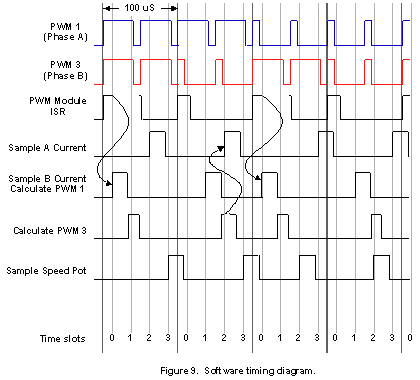After reset and before the main loop starts, internal processor functions and registers are initialized.  The PWM outputs are in a high impedance state, and pulled low to prevent current from flowing in any of the motor windings.  During the time when the motor current is zero, an ADC measurement of each motor phase is taken and saved as the measurement offset.  The PWM module is initialized to produce 20 kHz PWMs, and to generate an interrupt once every 2 PWM cycles.  Timer A is set up as a free running modulo counter with the same count modulus as the PWM ISR.  Thus, Timer A is synchronous to the PWM ISR period, and its four channels are configured to generate compare events at evenly spaced points throughout the PWM cycles.  The last step in the initialization routine is to start the PWM module and timer A.  Control is then passed to the MAIN routine, which is responsible for launching all non-time-critical background tasks that need to be performed.

Once the MR16 has been initialized properly, all aspects of controlling the motor are handled entirely via interrupt routines.  The current control loop for each motor coil runs at a 10 kHz sampling frequency, where the following tasks must be performed every 100 uS:

1. Sampling of the motor currents must be scheduled so as to not conflict with the PWM edges.
2. The speed potentiometer is sampled with the ADC.
3. Using the speed pot reading, a velocity profiler routine calculates a new commanded speed based on the specified acceleration-deceleration limit.
4. Using the newly calculated speed, two reference current values are fetched from a wavetable, one for each phase of the motor.
5. Phase A and phase B motor currents are sampled with the ADC.
6. The sampled motor current readings are processed and compared to the reference current for each phase.
7.  New PWM outputs are generated based on the error between the measured and reference current values.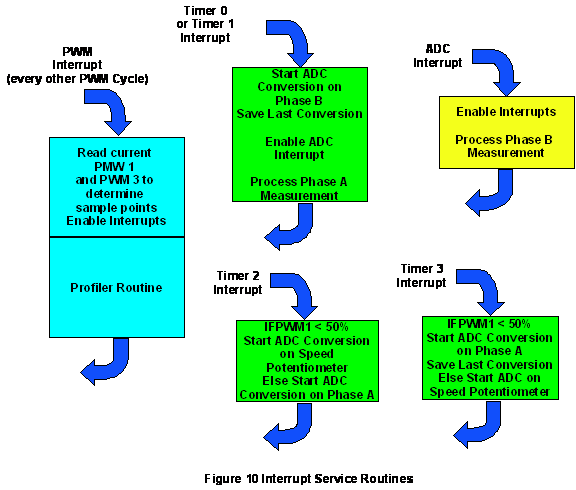An additional requirement placed on the control software is that the timing of the routines listed above must be “deterministic” in nature, i.e., the software can never loop waiting for some event to complete.  This guarantees that bandwidth is available to complete all the required control routines, as well as the non-time-critical background tasks.

To implement all the functions described above in the allotted time requires careful scheduling of events.  Fortunately, the ‘MR16 contains a rich complement of peripherals to assist with the above tasks.  Specifically, the on-board timers are an indispensable resource to the software to assist with staying on top of all the scheduling requirements.  To better appreciate the juggling required to dynamically schedule all the events, consider figure 9.  Each 100 uS period is divided into 4 evenly spaced time slots, which correspond to possible compare interrupts from timer channels 0-3.  Timer channels 0 and 1 are used for sampling the coil B current, and are configured to generate compare events at 25% and 75% respectively of the FIRST PWM cycle in the 100 uS period.  Likewise, timer channels 2 and 3 are used for sampling the coil A current, and are configured to generate compare events at 25% and 75% respectively of the SECOND PWM cycle in the 100 uS period.

The start of each 100 uS period is marked by an interrupt from the PWM module (one interrupt every two PWM cycles), and the PWM ISR is entered.  The first order of business in the ISR is to quickly examine the PWM pulse widths in order to sort out what tasks should be assigned to each of the time slots.  For example, if the PWM1 pulse width is greater than 50%, then the coil A current (which is determined by PWM1) should be scheduled for sampling in time slot 2 (timer channel 2 ISR) so as to not conflict with the PWM1 falling edge.  Conversely, if the PWM1 pulse width is less than 50%, the coil A current sample is scheduled for time slot 3 (timer channel 3 ISR).  The same rules apply to sampling the coil B current in time slot 0 or 1, depending on which one is furthest away from the PWM3 edge.  Once these tasks have been assigned, interrupts are re-enabled, and the remainder of the PWM module ISR is spent calculating new velocity information.  Motor speed can be specified as either a potentiometer ADC input, or via two digital inputs representing “step” and “direction”.

Referring again to figure 9, once interrupts have been re-enabled in the PWM module ISR, the next scheduled event is to sample the coil B current.  When the appropriate time slot interrupt occurs, the ISR initiates sampling of ADC2 (coil B current), and the existing reading in the ADC result register (which could either be the last speed pot reading, or the last coil A current reading) is saved.  Before exiting the timer ISR, the servo calculations required for updating the PWM1 pulse width are performed using the saved coil A current reading.

When the ADC interrupt signals that the conversion of coil B current is complete, execution is passed to the ADC ISR, where the servo calculations required for updating the PWM3 pulse width using the coil B current reading just acquired are performed.  During this time, interrupts must be re-enabled again, since it is possible that the next sampling of the coil A current may be scheduled for time slot 2, which would overlap this time.

If the coil A current sampling is NOT scheduled for time slot 2 (because of possible conflicts with the PWM1 falling edge), then it must be scheduled for time slot 3.  Since the pot sampling would not be affected by the PWM1 edge, it will then be scheduled for time slot 2 instead.  However, for the cases where the PWM1 edge could occur at or near time slot 3, then the sampling order of the coil A current and pot are reversed.

When performing the servo calculations required for updating the PWM values, the first step is to reconstruct the phase current from the ADC conversion.  The first step in reconstruction is to remove the offset that was measured and saved during initialization from the ADC conversion.  Next the measurement must be inverted if taken at the 75% point of the PWM cycle.  Recall from figure 8 that the voltage across the current sense resistor at the 75% ADC sample point is positive when the motor current is negative.  Finally, the measurement must be inverted once more to cancel the inversion from the sense amplifier (figure 8).

After reconstruction of the measured current, the commanded current is calculated from the wavetable as described earlier.  The table value is then used as the commanded current.  The measured current is subtracted from the commanded current resulting in the control system error signal.  Next the error signal is multiplied by a gain of 4 as shown in figure 6, and the result is output to the PWM module.  Note that the gain of 4 is easily implemented by shifting the error value two places to the left, which implies that the entire digital control loop is implemented without requiring any a multiply instructions.

Figure 10 shows a task summary for each interrupt service routine associated with the motor control task.  The entire control and profiler code takes approximately 65 microseconds to execute, leaving 35% of the processor bandwidth for other tasks.

Figures 11 and 12 show the motor current when the control has been configured for 10 microsteps per step and 50 microsteps per step respectively.  In each figure, a full cycle of the current and a zoomed view of the current waveform is shown.  Note that the zoomed waveforms have different amplitude scales, in order to better illustrate the current ripple due to the PWM action.  Operation with 50 microsteps per step results in a smoother current waveform as well as somewhat smoother operation at very slow speeds.  As the motor speed is increased, eventually the control system cannot regulate the current because the system saturates.  When saturation occurs, the control system automatically converts to a mode of operation where the current and voltage waveforms look similar to those of the square wave excitation mode.  Above this speed, no matter what control scheme is used, the motor current will begin to decrease, resulting in reduced torque.  Eventually, a speed is reached where the motor will not be able to keep up with the step rate and will stall.

As mentioned earlier, stepper motors are well suited for implementation on positioning systems.  The stepper motor control system described here with the use of CNC control software running on a PC was used to retrofit a milling machine with CNC capabilities.  The motors were microstepped at 10 microsteps per step offering theoretical resolution of 50 microinches per microstep, well in excess of the motor and machine accuracy.